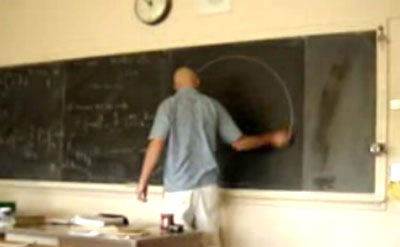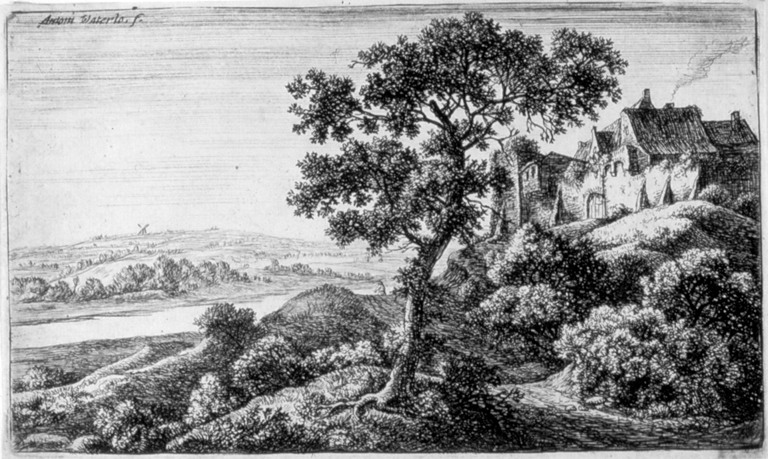# Drawing Machine

(diff) ← Older revision | Latest revision (diff) | Newer revision → (diff)

# Striving for the Pope's Commission

• Giotto's perfect circle.• Hand and Eye
• Legends: the high school teacher• linear apparatus. substructure.• Stroke recording apparatus. Two pulleys. Weights. Rotary encoders.

# Simultaneous Recording and Reproduction

• Simultaneous Location And Perversion (SLAP)

# Drawing Machine As Printmaking

• Harvey Moon
••• Antonie Waterloo
••# DynaCNC Drawing Machine

do a john henry with the dynaCNC## Pen Holder# Hanging Plotter

do a john henry with the hanging plotter

## Drawing Machine in Sketchbook# Articulated Arm

do a john henry with the disembodied hand.

## disembodied hand

Imprecision as painterly aesthetic.Aikon by Frédéric Fol Leymarie and Patrick Tresset.

# Thoughts

no such thing as perfect repetition. no matter what, there is noise in the system.

# Pantomime / Mirror

## GRBL1 fast

```\$0=7.023 (x, step/mm)
\$1=7.023 (y, step/mm)
\$2=7.023 (z, step/mm)
\$3=10 (step pulse, usec)
\$4=25400.000 (default feed, mm/min)
\$5=45720.000 (default seek, mm/min)
\$6=56 (step port invert mask, int:00111000)
\$7=25 (step idle delay, msec)
\$8=800.000 (acceleration, mm/sec^2)
\$9=0.050 (junction deviation, mm)
\$10=0.100 (arc, mm/segment)
\$11=25 (n-arc correction, int)
\$12=3 (n-decimals, int)
\$13=1 (report inches, bool)
\$14=1 (auto start, bool)
\$15=0 (invert step enable, bool)
\$16=0 (hard limits, bool)
\$17=1 (homing cycle, bool)
\$18=0 (homing dir invert mask, int:00000000)
\$19=400.000 (homing feed, mm/min)
\$20=10000.000 (homing seek, mm/min)
\$21=100 (homing debounce, msec)
\$22=1.000 (homing pull-off, mm)
```

## GRBL1 moderate

```fill in here
```

## grbl v 0.9a

```\$0=7.023 (x, step/mm)
\$1=7.023 (y, step/mm)
\$2=7.023 (z, step/mm)
\$3=500.000 (x v_max, mm/min)
\$4=500.000 (y v_max, mm/min)
\$5=500.000 (z v_max, mm/min)
\$6=10.000 (x accel, mm/sec^2)
\$7=10.000 (y accel, mm/sec^2)
\$8=10.000 (z accel, mm/sec^2)
\$9=10 (step pulse, usec)
\$10=20000.000 (default feed, mm/min)
\$11=56 (step port invert mask, int:00111000)
\$12=25 (step idle delay, msec)
\$13=0.050 (junction deviation, mm)
\$14=0.005 (arc tolerance, mm)
\$15=3 (n-decimals, int)
\$16=0 (report inches, bool)
\$17=1 (auto start, bool)
\$18=0 (invert step enable, bool)
\$19=0 (hard limits, bool)
\$20=0 (homing cycle, bool)
\$21=0 (homing dir invert mask, int:00000000)
\$22=25.000 (homing feed, mm/min)
\$23=250.000 (homing seek, mm/min)
\$24=100 (homing debounce, msec)
\$25=1.000 (homing pull-off, mm)
```